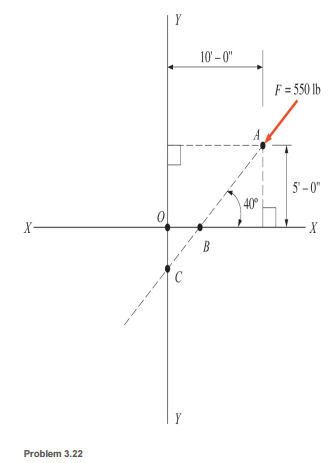### Create an Account

Home / Questions / Calculate the moment of the 550lb force about point O shown without using Varignons theore...

# Calculate the moment of the 550lb force about point O shown without using Varignons theorem Make a similar calculation using the theorem resolving the force into its X and Y components at point

Calculate the moment of the 550-lb force about point O shown without using Varignon’s theorem. Make a similar calculation using the theorem, resolving the force into its X and Y components at point A.Jun 26 2020 View more View LessSubscribe To Get Solution